CRR Tool
Capital Requirements Regulation (CRR)
Article 325g

# Article 325g - Own funds requirements for curvature risk

Status
In force
Selected consolidated version from
30/09/2021
Search all articles and keywords within this legal act

Article 325g

Own funds requirements for curvature risk

1.
Institutions shall perform the calculations laid down in paragraph 2 for each risk factor of the instruments subject to the own funds requirement for curvature risk, except for the risk factors referred to in paragraph 3.

For a given risk factor, institutions shall perform those calculations on a net basis across all the positions of the instruments subject to the own funds requirement for curvature risk that contain that risk factor.

2.
For a given risk factor k included in one or more instruments referred to in paragraph 1, institutions shall calculate the upward net curvature risk position of that risk factor (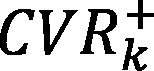) and the downward net curvature risk position of that risk factor (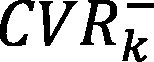) as follows: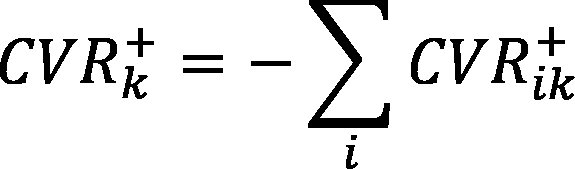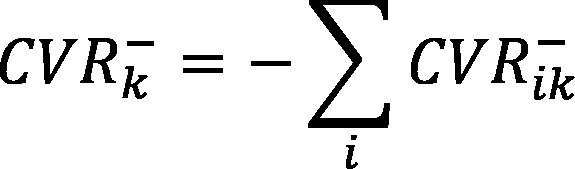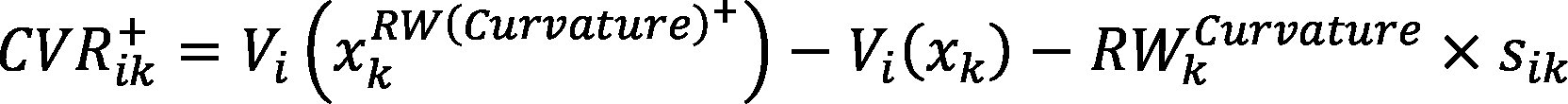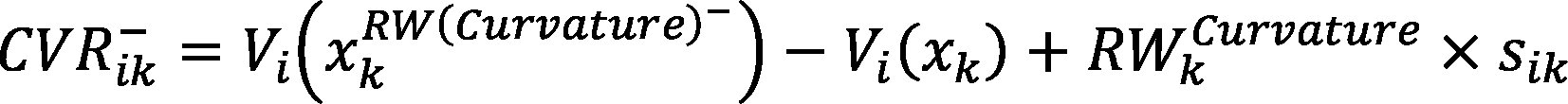where:

 i = the index that denotes all the positions of instruments referred to in paragraph 1 and including risk factor k;
 xk = the current value of risk factor k;
 Vi (xk ) = the value of instrument i as estimated by the pricing model of the institution based on the current value of risk factor k;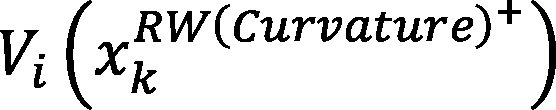= the value of instrument i as estimated by the pricing model of the institution based on an upward shift of the value of risk factor k;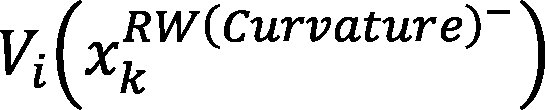= the value of instrument i as estimated by the pricing model of the institution based on a downward shift of the value of risk factor k;= the risk weight applicable to risk factor k determined in accordance with Section 6;
 sik = the delta sensitivity of instrument i with respect to risk factor k, calculated in accordance with Article 325r.
3.
By way of derogation from paragraph 2, for curves of risk factors that belong to the general interest rate risk (GIRR), credit spread risk (CSR) and commodity risk classes, institutions shall perform the calculations laid down in paragraph 6 at the level of the entire curve instead of at the level of each risk factor that belongs to the curve.

For the purposes of the calculation referred to in paragraph 2, where xk is a curve of risk factors allocated to the GIRR, CSR and commodity risk classes, sik shall be the sum of the delta sensitivities to the risk factor of the curve across all tenors of the curve.

4.
In order to determine a bucket-level own funds requirement for curvature risk, institutions shall aggregate, in accordance with the following formula the upward and downward net curvature risk positions, calculated in accordance with paragraph 2, of all the risk factors assigned to that bucket in accordance with Subsection 1 of Section 3: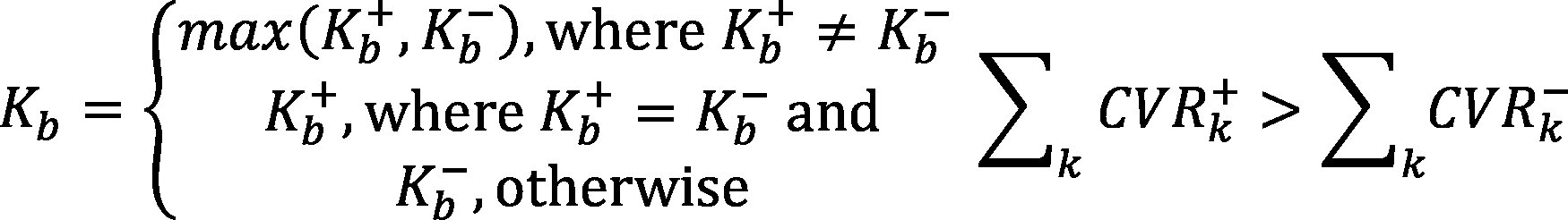where:

 b = the index that denotes a bucket of a given risk class;
 Kb = own funds requirements for curvature risk for bucket b;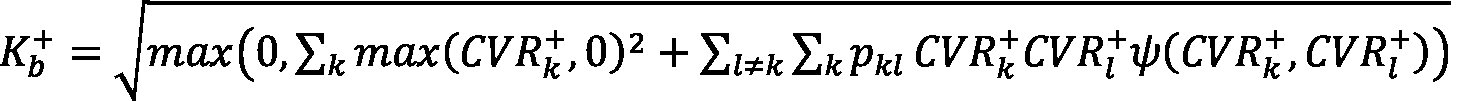;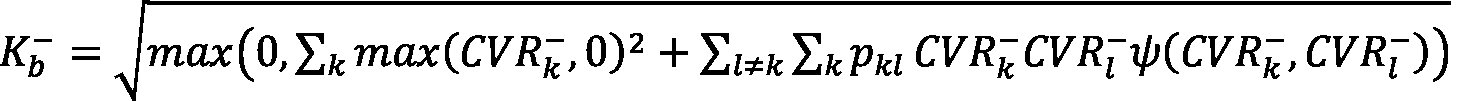;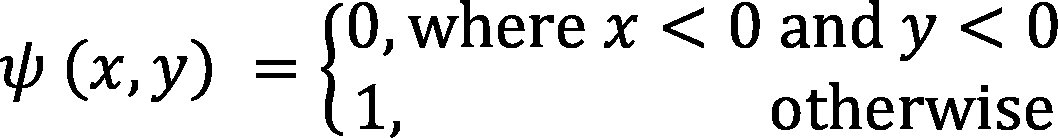;

 pkl = the intra-bucket correlations between risk factors k and l as prescribed in Section 6;
 k, l = the indices that denote all the risk factors of instruments referred to in paragraph 1 that are assigned to bucket b;
 () = the upward net curvature risk position;
 () = the downward net curvature risk position.
5.
By way of derogation from paragraph 4, for the bucket-level own funds requirements for curvature risk of bucket 18 of Article 325ah, of bucket 18 of Article 325ak, of bucket 25 of Article 325am and of bucket 11 of Article 325ap the following formula shall be used: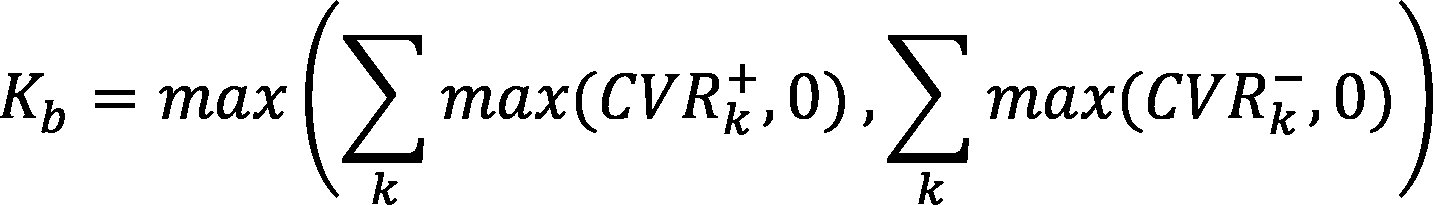6.
Institutions shall calculate the risk-class own funds requirements for curvature risk (RCCR) by aggregating all the bucket-level own funds requirements for curvature risk within a given risk class as follows: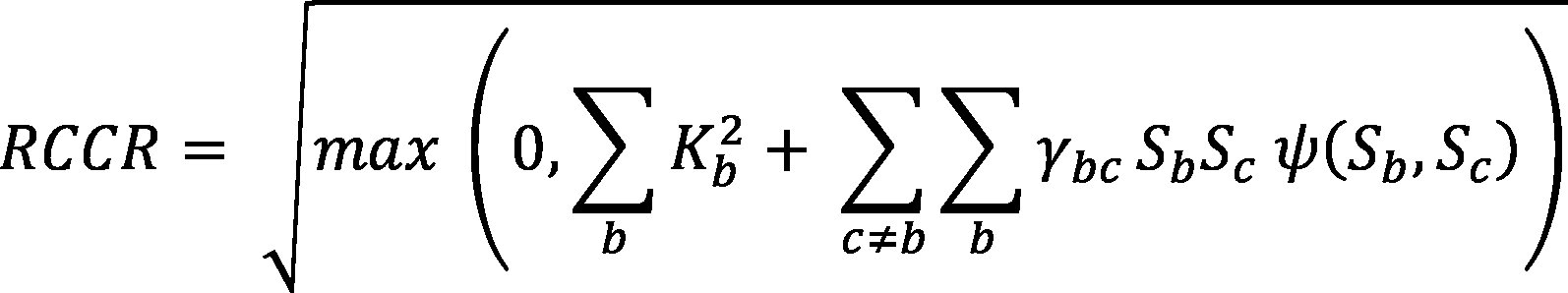where:

 b, c = the indices that denote all the buckets of a given risk class that corresponds to instruments referred to in paragraph 1;
 Kb = own funds requirements for curvature risk for bucket b;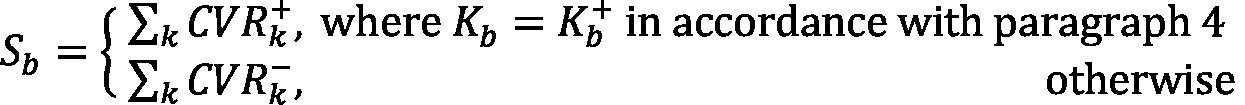;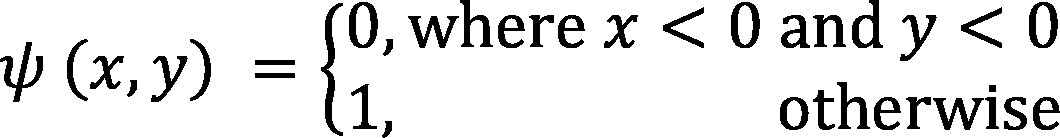;

 γbc = the inter-bucket correlations between buckets b and c as set out in Section 6.
7.
The own funds requirement for curvature risk shall be the sum of the risk class own funds requirements for curvature risk calculated in accordance with paragraph 6 across all risk classes to which at least one risk factor of the instruments referred to in paragraph 1 belongs.